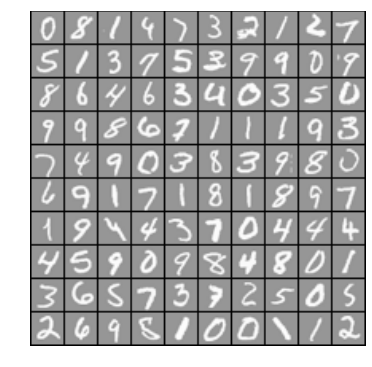## 3. Multi-Class Classification in Theano¶

The aim of this IPython notebook is to show some features of the Python Theano library in the field of machine learning. It has been developped by the LISA group at the University of Montreal (see: http://deeplearning.net/software/theano/). The notebook also relies on other standard Python libraries such as numpy, pandas and matplotlib.

To exemplify the use of Theano, this notebook solves the assignments of the Machine Learning MOOC provided by Coursera (see: https://www.coursera.org/learn/machine-learning) and performed in Stanford University by Andrew Ng (see: http://www.andrewng.org/).

The original MOOC assignments should to be programmed with the Octave language (see: https://www.gnu.org/software/octave/).The idea with this notebook is to provide Python developpers with interesting examples programmed using Theano.

This notebook has been developped using the Anaconda Python 3.4 distribution provided by Continuum Analytics (see: https://www.continuum.io/). It requires the Jupyter Notebook (see: http://jupyter.org/).

### 3.1 Logistic Regression¶

#### 3.1.1 Visualizing the data¶

In :
import theano
import numpy as np
import theano.tensor as T
from theano.tensor.nnet import sigmoid
import scipy.io as sio
data = sio.loadmat('data/ex3data1.mat')
X, Y = data['X'], data['y'].T
m = X.shape

In :
%matplotlib inline
import pandas as pnd
import matplotlib.pyplot as plt

def display_data(X, Y):
example_width = int(np.sqrt(X.shape))

# Compute rows, cols
m, n = X.shape
example_height = int(n / example_width)

# Compute number of items to display
display_rows = int(np.floor(np.sqrt(m)))
display_cols = int(np.ceil(m / display_rows))

# Between images padding
pad = 1

# Setup blank display
display_array = - np.ones((pad + display_rows * (example_height + pad),\
pad + display_cols * (example_width + pad)))

# Copy each example into a patch on the display array
# dataset contains 20 pixel by 20 pixel grayscale images of the digit
curr_ex = 0
for j in range(display_rows):
for i in range(display_cols):
if curr_ex >= m:
break
# Get the max value of the patch
max_val = max(abs(X[curr_ex]))
display_array[(pad + j * (example_height + pad)):(pad + j * (example_height + pad))+example_height,\
(pad + i * (example_width + pad)):(pad + i * (example_width + pad))+example_width] =\
np.reshape(X[curr_ex], (example_height, example_width)) / max_val
curr_ex += 1
if curr_ex >= m:
break

plt.figure(figsize=(8,6))
plt.axis('off')
plt.imshow(display_array.T, cmap="Greys_r")

df = pnd.DataFrame(Y.reshape((display_rows, display_cols)).T)
df = df.replace(10,0) # 0 is mapped to 10 in Y
print(df)

In :
rnd = np.random.permutation(m)[0:100]
sel = X[rnd,:] # 100 random digits
res = Y[rnd]
display_data(sel, res)

   0  1  2  3  4  5  6  7  8  9
0  0  8  1  4  7  3  2  1  2  7
1  5  1  3  7  5  3  9  9  0  9
2  8  6  4  6  3  4  0  3  5  0
3  9  9  8  6  7  1  1  1  9  3
4  7  4  9  0  3  8  3  9  8  0
5  6  9  1  7  1  8  1  8  9  7
6  1  9  4  4  3  7  0  4  4  4
7  4  5  9  0  9  8  4  8  0  1
8  3  6  5  7  3  7  2  5  0  5
9  2  6  9  5  1  0  0  1  1  2#### 3.1.2 Logistic Regression¶

In :
X1 = np.c_[np.ones(m), X] # Add intercept term to X
X1 = X1.T

# Choose some alpha and lambda values
alpha = 0.1
lambda_ = 0.1

num_labels = 10

all_theta = np.zeros((num_labels, X1.shape))

for j in range(num_labels):
print('Feature: %i' % (j+1))
# Init Theta and Run Gradient Descent
t = np.zeros(X1.shape)
theta = theano.shared(t,name='theta')

x = T.matrix('x')
y = T.vector('y')

h = sigmoid(T.dot(theta,x)) # 1.0 / (1.0 + T.exp(-T.dot(theta,x)))
reg = lambda_ * T.sum(theta[1:]**2) / 2 / m # regularization on theta
cost = -T.sum(y * T.log(h) + (1.0 - y) * T.log (1.0 - h))/m + reg
grad = T.grad(cost,theta)

train = theano.function([x,y],cost,updates = [(theta,theta-alpha*grad)])

num_iters = 100
for i in range(num_iters):
costM = train(X1, Y==(j+1))

all_theta[j] = theta.get_value()

# number of positive predictions where best prediction == Y-1
accuracy = np.sum(np.argmax(np.dot(all_theta,X1), axis=0) == (Y-1))/len(Y)
print('\nTrain Accuracy: %f' % accuracy)

Feature: 1
Feature: 2
Feature: 3
Feature: 4
Feature: 5
Feature: 6
Feature: 7
Feature: 8
Feature: 9
Feature: 10

Train Accuracy: 0.841600


### 3.2 Neural Networks¶

#### 3.2.1 Model representation¶

In :
# load precomputed network parameters
weights = sio.loadmat('data/ex3weights.mat')
theta1 = weights['Theta1']
theta2 = weights['Theta2']

# layer sizes
input_layer_size = X1.shape
hidden_layer_size = theta2.shape
output_layer_size = theta2.shape

print('input_layer_size: %i' % input_layer_size)
print('hidden_layer_size: %i' % hidden_layer_size)
print('output_layer_size: %i' % output_layer_size)

input_layer_size: 401
hidden_layer_size: 26
output_layer_size: 10


#### 3.2.2 Feedforward Propagation and Prediction¶

In :
x = T.matrix('x')

# first layer
y1 = 1 / (1 + np.exp(-np.dot(theta1,X1)))

# second layer
y2 = np.c_[np.ones(m), y1.T] # Add intercept term to y1
y2 = y2.T
y3 = 1 / (1 + np.exp(-np.dot(theta2, y2)))

In :
# number of positive predictions where best prediction == Y-1
accuracy = np.sum(np.argmax(y3, axis=0) == (Y-1))/len(Y)
print('Train Accuracy: %f' % accuracy)

Train Accuracy: 0.975200

In :
y1.shape, y2.shape

Out:
((25, 5000), (26, 5000))
In [ ]: